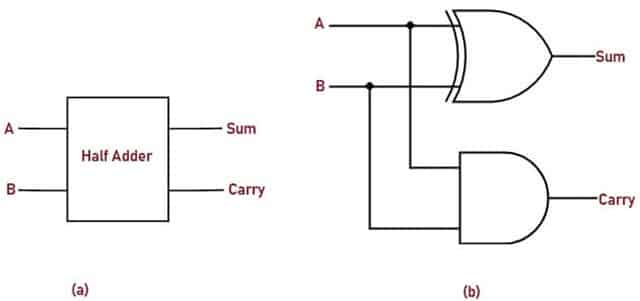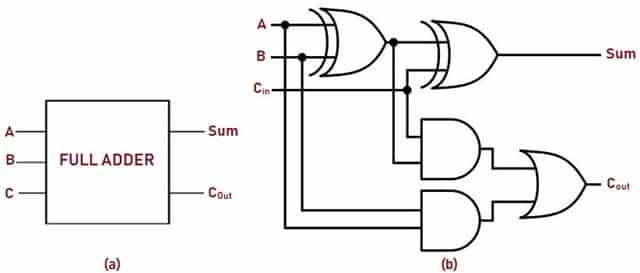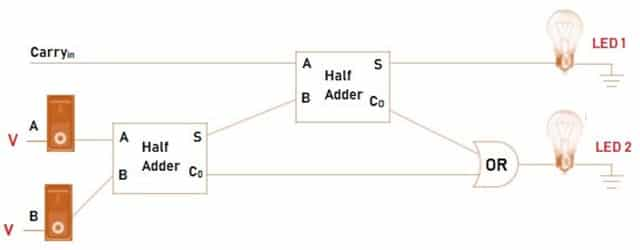Tuesday, October 26, 2021

# Adder – Classifications, Construction, How it Works and Applications

##### As the name suggests, Adder is used to add binary numbers. Adder circuit is basically a combinational logic circuit. It is a memory less circuit and performs an operation assigned to it logically by a Boolean expression. The output depends upon the present input at any given time.Fig. 1 – Block Diagram of Adder Circuit

##### For every clock pulse the bits are added simultaneously. There are different types of Parallel Adders. They are:
`Read More about Parallel Adders, How it Works, its Various Types, Applications & Advantages`

#####Fig. 2 – (a) Truth-Table of Half Adder Circuit (b) K-Map Simplification of Truth-Table

The Truth-Table representation for the inputs is as shown in the Fig. 2 – (a). From the Truth Table, it is clear that the Sum is 1 when the inputs are complementary. The Sum value is 0 when both the inputs are similar. This condition resembles the behavior of ‘XOR’ gate. If we analyse the Carry output, we conclude that when both the inputs are 1, Carry is 1. For rest of the conditions, its ‘0’. The condition for ‘Carry’ resembles the function of ‘AND’ gate. So, we can combine Sum and Carry outputs and implement Half Adder circuit using logic gates as shown in the Fig. 3(b).

Boolean expression for half Adder’s circuit obtained from K-Map simplification is:Fig. 3 – (a) Block Diagram (b) Circuit Diagram of Half Adder’s Circuit

When 3 bits need to be added, then Full Adder is implemented. It has three one-bit numbers as inputs, often written as A, B, and Cin where A and B are the operands and Cin is a carry bit from the previous less-significant stage. It adds 3 one bit numbers, out of which two of them are referred to as operands and the third one is referred to as a bit which is carried in. It produces 2 outputs, and they can be referred to as sum and carry output.

A Full Adder’s implementation is a bit difficult when compared to Half Adders. The difference between Half Adders and Full Adders is that the Full Adder’s circuit has three inputs and two outputs, whereas Half Adder’s circuit has only two inputs and two outputs. Here, A and B are the first two inputs and the third input is Carry-In (Cin). Once a Full Adder’s logic is designed, we can string eight of them together to create a byte-wide adder and cascade the carry bit from one Adder to the next.

Let us examine the possible outcomes of modulo addition for the inputs A, B, Cin

If A = 0, B = 0, Cin = 0 then Sum = 0, Carry-Out = 0

If A = 0, B = 0, Cin = 1 then Sum = 1, Carry-Out = 0

If A = 0, B =1, Cin = 0 then Sum = 1, Carry-Out = 0

If A = 0, B = 1, Cin = 1 then Sum = 0, Carry-Out = 1

If A = 1, B = 0, Cin = 0 then Sum = 1, Carry-Out = 0

If A = 1, B = 0, Cin = 1 then Sum = 0, Carry-Out = 1

If A = 1, B = 1, Cin = 0 then Sum = 0, Carry-Out = 1

If A = 1, B = 1, Cin = 1 then Sum = 1, Carry-Out = 1

From the Truth-Table, it is clear that the output Sum ‘S’ is XOR operation between the input A and the Half Adder’s Sum output with B and Cin inputs. The overall Carry-Out (Cout) will only be ‘True’ if any of the two inputs out of the three are HIGH.Fig. 4 – (a) Truth Table of Full Adder’s Circuit (b) K-Map Simplification of Truth Table

The Full Adder’s circuit can be implemented using the Truth Table and K-Map simplification. This circuit can be constructed by combining two Half Adders. Initially, first Half Adder’s circuit will be used to add inputs A and B to produce a partial sum. The second Half Adder’s circuit can be used to add input carry Cin to the sum produced by the first Half Adder, in order to get the final output ‘S’. If any one of the Half Adder’s logic produces a Carry, then there will be an output carry. So, Cout will be an OR function of both Half Adder’s Carry outputs. The larger logic diagrams can be implemented practically with the above mentioned Full-Adder logic circuit.

Fig. 5 shows Block Diagram and Circuit Diagram of Full Adder’s Circuit. With this type of construction, we can add two bits together, taking a carry from the next lower order of magnitude, and sending a carry to the next higher order of magnitude. In a computer, where multi-bit operation is involved, each bit must be represented by a Full Adder and must be added simultaneously. Thus, to add two 8-bit numbers, we will need 8 Full Adders which can be formed by cascading two of the 4-bit blocks.

#####Fig. 5 – (a) Block Diagram (b) Circuit Diagram of Full Adder’s Circuit

## How does Adder’s Circuit Work

To understand the working principle of an Adder circuit, Let us consider a Full Adder’s circuit implemented using 2 Half-Adders as shown in the Fig. 6. To verify the circuit, power connections are connected through ‘Switches’ to the inputs A and B. The output for each logic gate is displayed using LED.

Logic High = +5 V or Switch is “ON”

Logic Low = 0 V or Switch is “OFF”

The Truth-Table for Full Adders as shown in the Fig. 4 is verified experimentally.

Example:

If A = 00 = Switch is ‘OFF’

B = 0 = Switch is ‘OFF’

Cin = 1= Switch is ‘ON’

then Sum = 1 = LED 1 turns ‘ON’, Carry-Out = 0 = LED 2 turns ‘OFF’Fig. 6 – Working Principle of Full Adder’s Circuit using Two Half Adders

• A Full Adder’s circuit can be used as a part of many other larger circuits like Ripple Carry Adder, which adds n-bits simultaneously.
• The dedicated multiplication circuit uses Full Adder’s circuit to perform Carryout Multiplication.
• Full Adders are used in ALU- Arithmetic Logic Unit.
• In order to generate memory addresses inside a computer and to make the Program Counter point to next instruction, the ALU makes use of Full Adders.
• Full-Adders are a part of Graphics Processing Unit for graphics related applications.

• Half Adder’s design is very simple. It is the basic building block for single bit addition.
• Just with an inverter gate, Half-Adders can be converted to Half Subtractors.
• Full swing output can be obtained with Full-Adders.
• Power consumption is low.
• Speed is high.
• Robustness to supply voltage scaling.

• It does not incorporate (or take care of) previous carry for addition.
• To get rid of this problem, Full Adders are required which add three 1 bit.
• When Full Adders are used in a chain structure such as a Ripple Adder, the output drive capability is reduced.
```Also Read:
Logic Gates – Types, Working Principle, Application, Advantage
Transistor – Classification, Configuration, Applications, Advantages
PNP Transistor – Working Principle, Characteristics & Applications
T FLIP FLOP - Construction/ Design, Working Principle and Applications```V Rajeswari
V. Rajeswari ia a B.E(ECE), M.Tech(Embedded systems) and has work experience as Asst.Professor in Enggineering Colleges. She is an author, editor and partner at Electricalfundablog.
RELATED ARTICLES